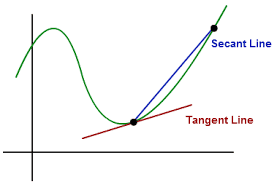## Friday, December 16, 2016

### Unizor - Derivatives - Parametric Curves

Notes to a video lecture on http://www.unizor.com

Differentiation
of Parametric Curves

Let's assume that we deal with some curve on the plane that is defined not as a graph of certain function that looks like y=f(x)(where x is abscissa and y - ordinate), but parametrically, where both coordinates are defined as functions of some parameter t.
An obvious example is a point moving on a plane, and its position {x;y} depends on a time parameter, so it can be described as {x(t);y(t)}, where both functions x(t) and y(t) are given and differentiable.
So, one independent parameter describes both coordinates through these two function.

Our task is to find an equation that describes the tangential line to this curve at some point{x0;y0} that corresponds to a parameter value t=t0, that is x0=x(t0) and y0=y(t0).

Geometrically speaking, a tangential line to a sufficiently smooth curve at some point is a limit of a secant line that intersects our curve at point where a tangential line should be and another point close to it, when that other point is getting closer and closer to the point of tangency.Generally, a straight line that goes through point {x0;y0} has a point-slope equation
y−y0 = m·(x−x0)
So, all we have to determine in the equation for a tangential line that goes through point{x0;y0} is its slope m.

Since our tangential line is a limit of a secant, we can assume that the slope of a tangential line is the limit of a slope of a secant as the other point of secant's intersection with our curve is getting infinitesimally close to point {x0;y0}.

Consider a point of tangency{x0=x(t0);y0=y(t0)} and give an increment to parameter t from its value t0 to value t0+Δt.
The new point on a curve that corresponds to an incremented value of parameter t will be
{x1=x(t0+Δt);y1=y(t0+Δt)}

A secant that intersects our curve at points {x0;y0} and{x1;y1} has a slope equal to
m = (y1−y0)/(x1−x0)
which can be transformed into
m = Δy/Δx
where Δy=y(t0+Δt)−y(t0)
and Δx=x(t0+Δt)−x(t0)

Obviously, we want to express the limit of this expression for slope m as Δt→0 in terms of derivatives Dt[x(t)] and Dt[y(t)].
For this we can transform it into
m = y/Δt)/x/Δt)
from which follows that for Δt→0
m→m0=Dt=t0[y(t)] /Dt=t0[x(t)]
where derivatives are taken at point t=t0.

Example

A unit circle with a center at the origin of coordinates can be described parametrically with an angle θ from the positive direction of the X-axis to a radius to a point on a circle being a parameter. Let's use the radian measure of this angle.

Any point on a circle with coordinates {x;y} can be described through functions:
x=cos(θ),
y=sin(θ).

Let's choose a point that corresponds to a parameter value θ=π/4 and determine the equation of a tangential line at this point.
First of all, determine the coordinates of a point of tangency:
x=cos(π/4)=√2/2
y=sin(π/4)=√2/2
Now the slope should be equal to a ratio of derivatives of functions y(θ) and x(θ) at point with parameter θ=π/4:
Dθ=π/4[y(θ)]=Dθ=π/4[sin(θ)]=
cos(π/4) = √2/2
Dθ=π/4[x(θ)]=Dθ=π/4[cos(θ)]=
−sin(π/4) = −√2/2

The slope m of our tangential line is a ratio of the two values above:
m = (√2/2)/(−√2/2) = −1

So, the equation of our tangential line at point defined by parameter θ=π/4 in point-slope form is
y−√2/2 = −1·(x−√2/2)
or, in a standard form,
y = −x + √2